# Simple Vibration Neutraliser Notes - Mechanical Engineering

## Mechanical Engineering: Simple Vibration Neutraliser Notes - Mechanical Engineering

The document Simple Vibration Neutraliser Notes - Mechanical Engineering is a part of Mechanical Engineering category.
All you need of Mechanical Engineering at this link: Mechanical Engineering

Dynamic Vibration Absorbers

Basic Concept

Dynamic Vibration Absorbers (DVA) are based on the concept of attaching a secondary mass to a primary vibrating system such that the secondary mass dissipates the energy and thus reduce the amplitude of vibration of the primary system.

There are many application of DVA, A few are noted below:

• vibration control of transmission cables
• control of torsional oscillation of crankshaft
• control of rolling motion of ships
• chatter control of cutting tools
• control of noise in aircraft cabin
• vibration control of hand held devices

DVAs are generally of three types

• Vibration Neutralizer :

Here, a secondary mass is connected to the primary using a spring element.

• Auxiliary Mass Damper :

Here the secondary mass is connected to the primary by a damper/dashpot.

• Dynamic Vibration Absorber:

A general case where both spring and damper are used to connect the secondary mass, with the primary system.

Model of a Simple Vibration Neutralizer

The primary system is assumed to be an undamped single-degree-of-freedom system of mass mand stiffness k1. The secondary system (the neutralizer) consists of mass m2 attached to the primary using a spring of stiffness k2 . The primary system is subjected to a harmonic excitation as shown in figure 16.1.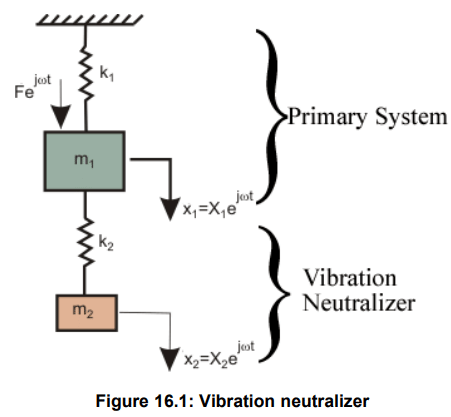The responses of the masses from their respective equilibrium positions are denoted as x1 for m1 and x2 for m2 . The equations of motion for the masses can be written as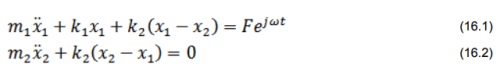In the steady state, the solutions of the governing equations are assumed to be x1 = x1ejwt and x2 = x2ejwt . Substituting these in eqns (16.1) and (16.2), we get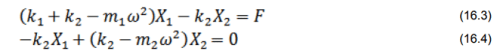Solving eqns. (16.3) and (16.4), we obtain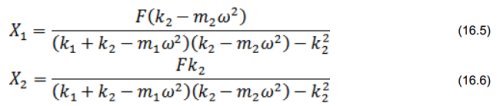From eqns. (16.5) and (16.6), you may note that if the secondary system is tuned to the excitation frequency, i.e., its natural frequency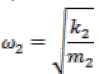is made equal to , then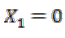and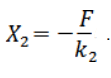This implies that the primary system comes to rest, after tuning.

Design Considerations

• It should be noted that a tuned neutralizer makes the response of the primary system zero only at one frequency, namely, w2. So, the application of such a neutralizer is very much limited.
• Eventhough the tuned mass damping system could successfully neutralize the vibration response of the primary system when the excitation frequency is w2; it also introduces two new resonating frequencies to the original system. Hence, care should be taken such that the two new frequencies are kept sufficiently away from the expected excitation frequency.
• From equation 16.6, you may note that at w=w2 , displacement of the secondary mass,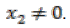. In fact in many system, there is a constraint on maximum permissible value of x2. This is known as rattle space.
The document Simple Vibration Neutraliser Notes - Mechanical Engineering is a part of Mechanical Engineering category.
All you need of Mechanical Engineering at this link: Mechanical EngineeringUse Code STAYHOME200 and get INR 200 additional OFF

Track your progress, build streaks, highlight & save important lessons and more!

,

,

,

,

,

,

,

,

,

,

,

,

,

,

,

,

,

,

,

,

,

;